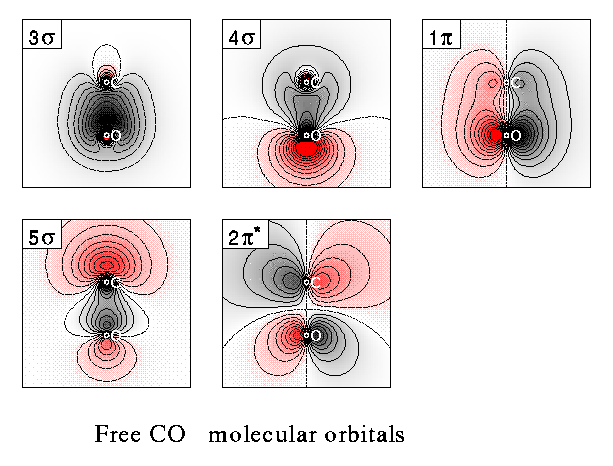# Electron Density of $$\sigma$$ and $$\pi$$ Bonds

Skills to Develop

• Explain the hybrid atomic orbitals used in sigma and pi bonding.
• Describe the shapes and electron density distributions of the sigma and pi bonds.
• Apply valence bond and molecular orbital theory to explain sigma and pi bonds.

## Electron Density of Sigma and Pi Bonds

Years ago, a student in my class asked me if I could write a computer simulation to plot the electron density of Sigma and Pi Bonding and their Anti bonding molecular orbitals.

He did have a chance to see the results of an approximate simulation.

The electron density should be displayed in a 3-dimensional space, but the computer screen only provides a flat plane to show the results. Suppose we cut the 3-dimensional space into two halves, the section looks like the plot. Thus, the plots are not projections, but cross sections.

Since my simulation runs in a DOS environment, it cannot be shown on the web pages. However, here are some excellent diagrams calculated using quantum mechanical wave functions.

### Electron Densities of O2 Molecules

The following diagram shows the bonding orbitals of oxygen molecules. For oxygen, the electronic configuration is

1sg2 1su2 2sg2 1pu4 1pg2 2su

However, the diagram seems to have the molecular orbitals labeled as

2sg2 2su2 3sg2 1pu4 1pg2 3su

The 2sg orbital is derived from the 2s atomic orbitals of oxygen, and 3sg orbital is derived from the 2p atomic orbitals. The p orbitals are derived from the p atomic orbitals.### Electron Densities of CO Molecules### What Should You Be Able to Do After You Have Seen These Plots?

1. You should know what sigma and pi bonds are. What atomic orbitals (AO) are used to get hybrid AOs? What hybrid AOs are used for the sigma and pi bonding? Draw a picture showing the "shape" of the hybrid atomic orbitals used. Show how the atomic orbitals overlap to form the sigma and pi bonds.
2. You see the electron density of the Sigma and Pi molecular orbitals. These orbitals are made up using a Linear Combination of Atomic Orbitals (LCAO). From these plots, draw a curve through areas where the density of dots is about the same. These curves represent the "shapes" of the molecular orbitals.
3. You should understand that the sigma bond is symmetrical along the bonding axis, but the Pi bond has a nodal plane that contains the bond axis.
4. Review the lecture material on Molecular Orbital. Draw energy levels of the MO relative to the AO.

## Confidence Building Questions

1. What are the atomic orbitals (AO) used to get the sp2 hybrid orbitals?

Hint: One s and two p atomic orbitals.

2. In the compound $$\ce{HCHO}$$, what hybrid AO's are used by the $$\ce{C}$$ atom?

Hint: The sp2 hybrid orbitals of $$\ce{C}$$ are involved.

Discussion -
The $$\ce{C}$$ utilizes sp2 hybrid orbitals for the sigma bonds.

H
\
C = O;  but a p orbital is used for the pi bond between C and O.
/
H

3. What hybrid atomic orbitals are used for the $$\ce{C}$$ in $$\ce{CCl4}$$?

Hint: The sp4 hybrid orbitals of $$\ce{C}$$ are involved.

Discussion -
The sp3 hybrid AO's have points towards the vertices of a tetrahedron.

4. What is the value of the bond angles around the $$\ce{C}$$ atom in $$\ce{CH3OH}$$?

Hint: The bond angle is 109.5 degrees.

Discussion -
The tetrahedral arrangement of the bonds around $$\ce{C}$$ in methanol gives an average bond angle of 109.5°.

5. What is the bond order between carbon atoms in the benzene molecule?

Hint: The bond order is 1.5 in benzene.

Discussion -
Three double bonds spread over 6 $$\ce{C-C}$$ bonds. Thus, the bond order is 1.5.